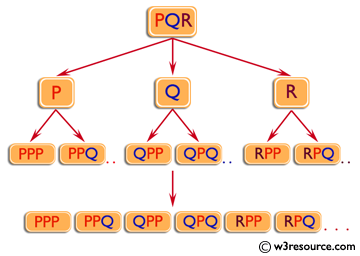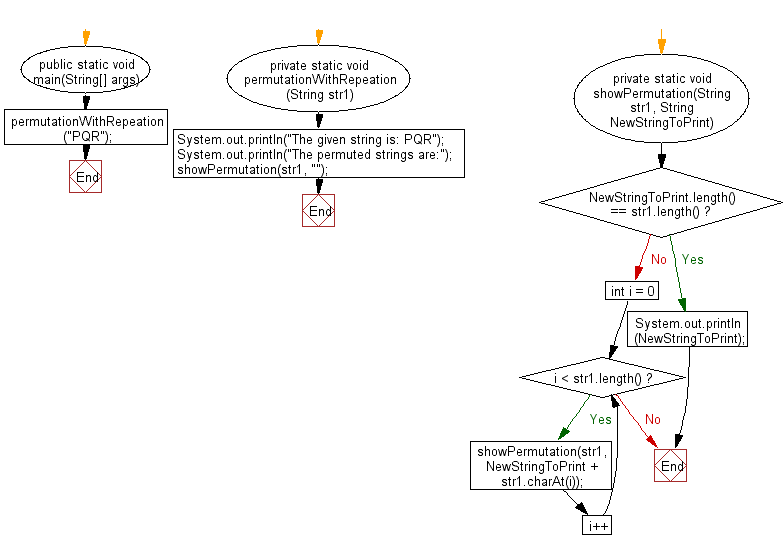﻿ Java - Print all permutations of a specified string

# Java: Print all permutations of a specified string with repetition

## Java String: Exercise-35 with Solution

Write a Java program to print all permutations of a given string with repetition.

Visual Presentation:Sample Solution:

Java Code:

``````// Import necessary Java utilities.
import java.util.*;

// Define a class named Main.
public class Main {

// The main method to start the execution of the program.
public static void main(String[] args) {
// Call the permutationWithRepeation method with the given string "PQR".
permutationWithRepeation("PQR");
}

// Method to find permutations with repetition of characters.
private static void permutationWithRepeation(String str1) {
// Print the given string.
System.out.println("The given string is: PQR");
// Print the message for the permuted strings.
System.out.println("The permuted strings are:");
// Call the showPermutation method to display the permutations.
showPermutation(str1, "");
}

// Recursive method to generate and display permutations.
private static void showPermutation(String str1, String newStringToPrint) {
// If the length of the new string equals the length of the original string, print the new string.
if (newStringToPrint.length() == str1.length()) {
System.out.println(newStringToPrint);
return;
}

// Iterate through the characters of the original string.
for (int i = 0; i < str1.length(); i++) {
// Recursively call showPermutation to append characters and generate permutations.
showPermutation(str1, newStringToPrint + str1.charAt(i));
}
}
}
```
```

Sample Output:

```The given string is: PQR
The permuted strings are:
PPP
PPQ
PPR
PQP
PQQ
PQR
PRP
PRQ
PRR
QPP
QPQ
QPR
QQP
QQQ
QQR
QRP
QRQ
QRR
RPP
RPQ
RPR
RQP
RQQ
RQR
RRP
RRQ
RRR
```

Flowchart:Java Code Editor:

Improve this sample solution and post your code through Disqus

What is the difficulty level of this exercise?

Test your Programming skills with w3resource's quiz.

﻿